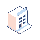• Preiskalkulation

# how to calculate difference bitween two dates ?

Hi, in my object I have two date fields: date1:fields.date('date 1'), date2:fields.date('date 2'),

I want to retrieve the difference of that 2 fields I tried this : date2 - date1 but I got this error : TypeError: unsupported operand type(s) for -: 'str' and 'str'

2 Kommentareto do this, the first you must convert into date format (use lib datetime: datetime.date(year, month, day)). Then you can use operator '-' in this case. Refer: http://docs.python.org/2/library/datetime.html

##### 17 Antworten

Hi mos, You need to do some python level stuffs with dates for getting exact date difference , i will explain with an example

``````from datetime import datetime
fmt = '%Y-%m-%d'
from_date = '2013-09-01'     -> Take your date field1
to_date = '2013-09-31'       -> Take your date field2
d1 = datetime.strptime(from_date, fmt)
d2 = datetime.strptime(to_date, fmt)
daysDiff = str((d2-d1).days)          -> will gives you 29 days
daysDiff = str((d2-d1).days + 1)      -> will gives you 30 days
``````

Hope it helps you ........ Thanks

12 Kommentarehi nishad thanks for your answer i want to calculate it automatically and show in readonly=True field so can you please provide me some extra detail for calculate duration

Define two date fields(from date , to date) & one functional field(difference_days) to keep the difference days. Do implement the date difference calculation steps in your function and return the value . A functional field in openerp will return a readonly = True value by default. If you want to save data in to DB then use store=True parameter while defining functional field.

my field is 'date_s':fields.datetime('Start Date'), 'date_e':fields.datetime('End Date'), 'duration':fields.function(_get_days, string='Duration', store=True), and my function is this def _get_days(self, cr, uid, ids, field_name, args, context=None): res = {} for date in self.browse(cr, uid, ids, context=context): res[date.id] = ((date.date_s-date.date_e).days) or False return res but it give me error like this res[date.id] = ((date.date_s-date.date_e).days) or False TypeError: unsupport

You need to include 'from datetime import datetime' in the import section and then do a date formatting using 'datetime.strptime(from_date, fmt)' for each date fields where fmt = '%Y-%m-%d' , because we are doing a conversion from openerp datetime format to native python format and then calculating the difference . Do one by one as i shown in my source code instead of doing it in single step.

i correct my function but still i got error like this data_string[found.end():]) ValueError: unconverted data remains: 10:52:57 and my function is def _get_days(self, cr, uid, ids, field_name, args, context=None): res = {} for date in self.browse(cr, uid, ids, context=context): fmt = '%Y-%m-%d' date.date_s = datetime.strptime(date.date_s, fmt) date.date_e = datetime.strptime(date.date_e, fmt) res[date.id] = ((date.date_s-date.date_e).days) or False return res

This is because your fields are of type' datetime' , instead use normal 'date' in openerp.

def _get_days(self,cr,uid,ids,field_name, arg ,context=None):
res = {}, fmt = '%Y-%m-%d', for object in self.browse(cr, uid, ids, context=context): res[object.id] = {'diff_days':0, } from_date = object.date_a, to_date = object.date_b, d1 = datetime.strptime(from_date, fmt), d2 = datetime.strptime(to_date, fmt) ,daysDiff = str((d2-d1).days), res[object.id]['diff_days'] = daysDiff , return res

'date_a':fields.date("Date1"), 'date_b':fields.date("Date2"), 'diff_days':fields.function(_get_days,string="Diff days", multi='sums',store=True),

Hi,

date = fields.Date.from_string(old_date)

worked_days = (fields.date.today() - date).days

Regards,Use timedelta object form python

from datetime import timedelta

https://docs.python.org/2/library/datetime.html#timedelta-objects